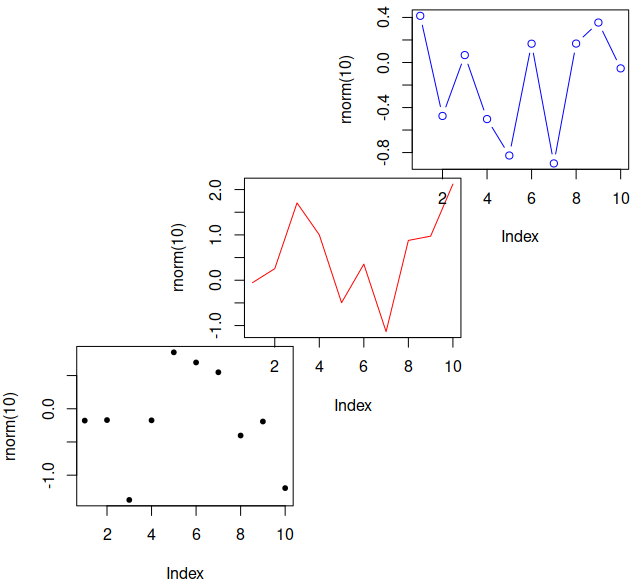## Quick Start

screen() 命令用于选取作图的图形区，erase.screen 通过用背景色填充来擦除一个图形区，close.screen 移除一个或多个图形区。

split.screen() 里的例子（有修改）：

set.seed(1234)

split.screen(c(2, 1))
##  1 2
##  1 2
screen()
##  1
##  1
split.screen(c(1, 2), screen = 2)
##  3 4
##  3 4
screen()
##  3
##  3

screen(2)
par(mar = c(4, 4, 1, 0) + .1)
plot(rnorm(20), pch = 17)

screen(3, new = FALSE)
par(mar = c(4, 4, 1, 0) + .1)
plot(rnorm(10), col = 'red', pch = 18, cex = 1.5, type = "b")

screen(4, new = FALSE)
par(mar = c(4, 4, 1, 0) + .1)
plot(rnorm(10), col = 'blue', pch = 20, cex = 1.5, type = "b")

x <- density(rnorm(30))
screen(1)
par(mar = c(4, 4, 1, 0) + .1)
plot(x, type = "n", main = "")
polygon(x, col = "violetred")

(动画好像没法展示出来，我只能文字描述了…)

## figs 参数

split.screen() 命令接受三个参数，split.screen(figs, screen, erase = TRUE)，后两个很好理解。figs 参数就很强大了。上面的例子里都是接受一个形如 par(mfrow) 形式的参数直接指定行列数，但是这个参数还接受通过一个矩阵来定义多个图形设备的方式。具体形式是 N 行 4 列，每一行四个数字分别表示图形设备的左、右、下、上四个顶点的位置，这样就能精确控制每个图形设备的大小和位置。比如：

split.screen(rbind(c(0, .5, 0, .5),
c(.25, .75, .25, .75),
c(.5, 1, .5, 1)))
screen(1)
plot(rnorm(10), col = "black", pch = 20, )
screen(2, new = FALSE)
plot(rnorm(10), col = "red", type = "l")
screen(3, new = FALSE)
plot(rnorm(10), col = "blue", type = "b")figs